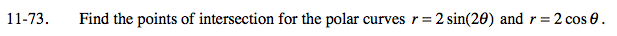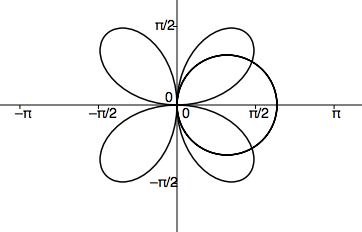### Home > PC > Chapter 11 > Lesson 11.2.1 > Problem11-73

11-73.Set the equations equal to each other (because r = r).

Divide both sides by 2.

Apply the Double Angle Identity for sine.

Set the equation equal to 0 and factor.

Use the Zero Product Property to solve for θ.

Use your values for θ to solve for r.

Verify your answers and that you found all points of intersection by graphing.